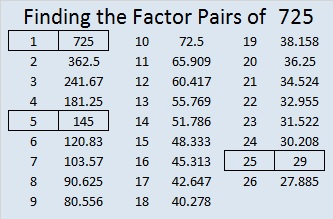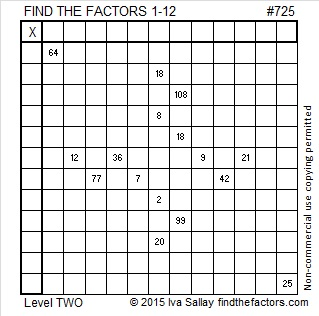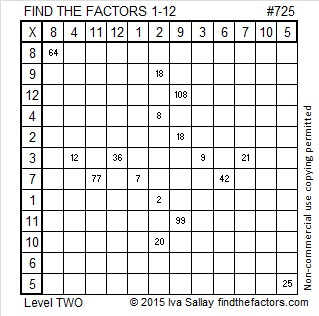# 725 and Level 2

• 725 is a composite number.
• Prime factorization: 725 = 5 x 5 x 29, which can be written 725 = (5^2) x 29
• The exponents in the prime factorization are 2 and 1. Adding one to each and multiplying we get (2 + 1)(1 + 1) = 3 x 2  = 6. Therefore 725 has exactly 6 factors.
• Factors of 725: 1, 5, 25, 29, 145, 725
• Factor pairs: 725 = 1 x 725, 5 x 145, or 25 x 29
• Taking the factor pair with the largest square number factor, we get √725 = (√25)(√29) = 5√29 ≈ 26.925824.Give this Level 2 puzzle a try!Print the puzzles or type the solution on this excel file: 12 Factors 2015-12-28

—————————————————————————————————

Perhaps, you would like to know some other facts about the number 725:

725 can be expressed as the sum of consecutive numbers five different ways:

• 362 + 363 = 725; that’s 2 consecutive numbers.
• 143 + 144 + 145 + 146 + 147 = 725; that’s 5 consecutive numbers.
• 68 + 69 + 70 + 71 + 72 + 73 + 74 + 75 + 76 + 77 = 725; that’s 10 consecutive numbers.
• 17 + 18 + 19 + . . . + 29 + . . . + 39 + 40 + 41 = 725; that’s 25 consecutive numbers.
• 11 + 12 + 13 + . . . + 25 + . . . + 37 + 38 + 39 = 725; that’s 29 consecutive numbers.

725 is also the sum of the eleven prime numbers from 43 to 89.

The factors in one of its factor pairs, 25 x 29, are both 2 numbers away from their average, 27, so 725 is just 4 numbers away from perfect square 27² = 729 . Thus, 25 x 29 =  (27 – 2)(27 + 2) = 27² – 2² = 729 – 4 = 725.

725 is the sum of two squares three different ways:

• 26² + 7² = 725
• 25² + 10² = 725
• 23² + 14² = 725

Because ALL of its prime factors have a remainder of one when divided by four, 725 is the hypotenuse of primitive Pythagorean triples:

• 364-627-725 which was calculated using 2(26)(7), 26² – 7², 26² + 7²
• 333-644-725 which was calculated using 23² – 14², 2(23)(14), 23² + 14²

It is also the hypotenuse of FIVE other Pythagorean triples.

• 85-720-725
• 120-715-725
• 203-696-725
• 435-580-725
• 500-525-725

725 is a palindrome in two bases:

• 505 BASE 12; note that 5(144) + 0(12) + 5(1) = 725.
• PP BASE 28 (P = 25 base 10); note that 25(28) + 25(1) = 725.

—————————————————————————————————## 3 thoughts on “725 and Level 2”

1.nikirath

hi Iva , Hope you are doing good. I was curious to know how you have setup your website that it comes up on google search , when I try and search mine , it doesnt. Did you do something special to set that up. Please let me know 🙂

Thanks ,
Nikita

•ivasallay

So far I haven’t done anything except tag my posts. The following post from wordpress caught my eye last year, I ran the diagnostics and FAILED but still haven’t applied the advice yet. I hope you find it helpful! https://cloudladder.wordpress.com/2015/05/17/is-your-website-being-slighted-by-google-and-bing/

•nikirath

Thank you Iva 🙂

This site uses Akismet to reduce spam. Learn how your comment data is processed.